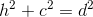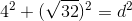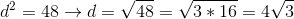# GMAT Math : Calculating the diagonal of a cube

## Example Questions

### Example Question #7 : Cubes

What is the length of the diagonal of a cube if its side length is?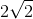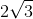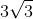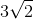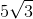Explanation:

The diagonal of a cube extends from one of its corners diagonally through the cube to the opposite corner, so it can be thought of as the hypotenuse of a right triangle formed by the height of the cube and the diagonal of its base. First we must find the diagonal of the base, which will be the same as the diagonal of any face of the cube, by applying the Pythagorean theorem: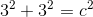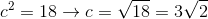Now that we know the length of the diagonal of any face on the cube, we can use the Pythagorean theorem again with this length and the height of the cube, whose hypotenuse is the length of the diagonal for the cube: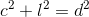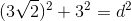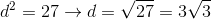### Example Question #1 : Calculating The Diagonal Of A Cube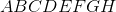is a cube and facehas an area of. What is the length of diagonal of the cube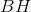Explanation:

To find the diagonal of a cube we can apply the formula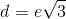, whereis the length of the diagonal and where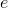is the length of an edge of the cube.

Since we are given an area of a face of the cube, we can find the length of an edge simply by taking its square root.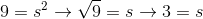Here the length of an edge is 3.

Thefore the final andwer is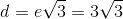.

### Example Question #9 : Cubes

What is the length of the diagonal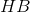of cube, knowing that facehas diagonal equal to?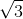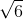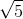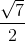Explanation:

To find the length of the diagonal of the cube, we can apply the formula, however, we firstly need to find the length of an edge, by applying the formula for the diagonal of the square.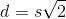whereis the diagonal of face ABCD, and, the length of one of the side of this square.

The length ofmust be, which is the length of the edges of the square.

Therefore we can now use the formula for the length of the diagonal of the cube: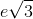, whereis the length of an edge.

Since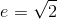, we get the final answer.

### Example Question #10 : Cubes

A given cube has an edge length of. What is the length of the diagonal of the cube?

Not enough information provided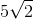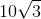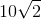Explanation:

The diagonalof a cube with an edge lengthcan be defined by the equation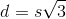. Given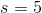in this instance,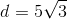.

### Example Question #1 : Calculating The Diagonal Of A Cube

A given cube has an edge length of. What is the length of the diagonal of the cube?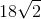Not enough information provided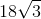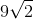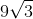Explanation:

The diagonalof a cube with an edge lengthcan be defined by the equation. Given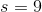in this instance,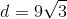.

### Example Question #2 : Calculating The Diagonal Of A Cube

A given cube has an edge length of. What is the length of the diagonal of the cube?None of the aboveExplanation:

The diagonalof a cube with an edge lengthcan be defined by the equation. Given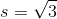in this instance,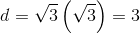.

### Example Question #3 : Calculating The Diagonal Of A Cube

If a cube has a side length of, what is the length of its diagonal?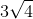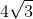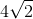Explanation:

The diagonal of a cube is the hypotenuse of a right triangle whose height is one side and whose base is the diagonal of one of the faces. First we must use the Pythagorean theorem to find the length of the diagonal of one of the faces, and then we use the theorem again with this value and length of one side of the cube to find the length of its diagonal: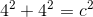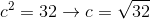So this is the length of the diagonal of one of the faces, which we plug into the Pythagorean theorem with the length of one side to find the length of the diagonal for the cube: